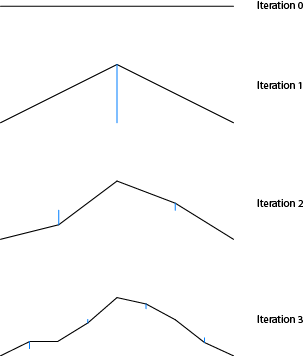Aschenblog: Thoughts on Code and Fabrication

# 2D Fractal Terrain Generation

A while ago I discovered a simple algorithm to generate infinite fractal terrain. One application for this is to produce mountain-like backgrounds for 2D side-scrolling video games.This is called the midpoint displacement algorithm. It works by recursively breaking a line into smaller and smaller segments and at each step changing the Y-value up or down by a random amount. The amount of change is reduced by some amount at each step to produce a rough or smooth looking mountain scape.

The blue lines (pictured right) indicate the location and amount of displacement from the previous iteration.

Here is an outline of the algorithm:

1. Find the midpoint for the line segment
2. Assign the midpoint to the average of the endpoints (L + R) / 2
3. Generate a random number between -1 to 1 and multiply by the displacement value. Add this to the midpoint value.
4. Recursively subdivide this line and reduce the displacement value by a fixed amount (a roughness parameter)
5. Repeat previous until fractal is sufficiently detailed

Note that the roughness parameter needs to greater than zero and less than one. Higher values result in rougher terrain and lower values result in smoother terrain. Typical values may range between 0.5 to 0.75 and depend on depth of recursion.

Also, note that you will have to determine what sufficiently detailed means. Arrays sized 2N + 1 are typically used to represent terrain height values. A good stopping point is when all array indicies are populated with values.

Let’s take a look at a Javascript implementation of the algorithm above.

The if block on line two prevents infinite recursion. Also note, that we define a global array of floating point values called terrain_array. All values in the array are initialized to zero. We also defined a global variable for roughness.

Here is the code in action:

I added animation to show how easy it is to adapt this algorithm for side scrolling action. The code is available on github.